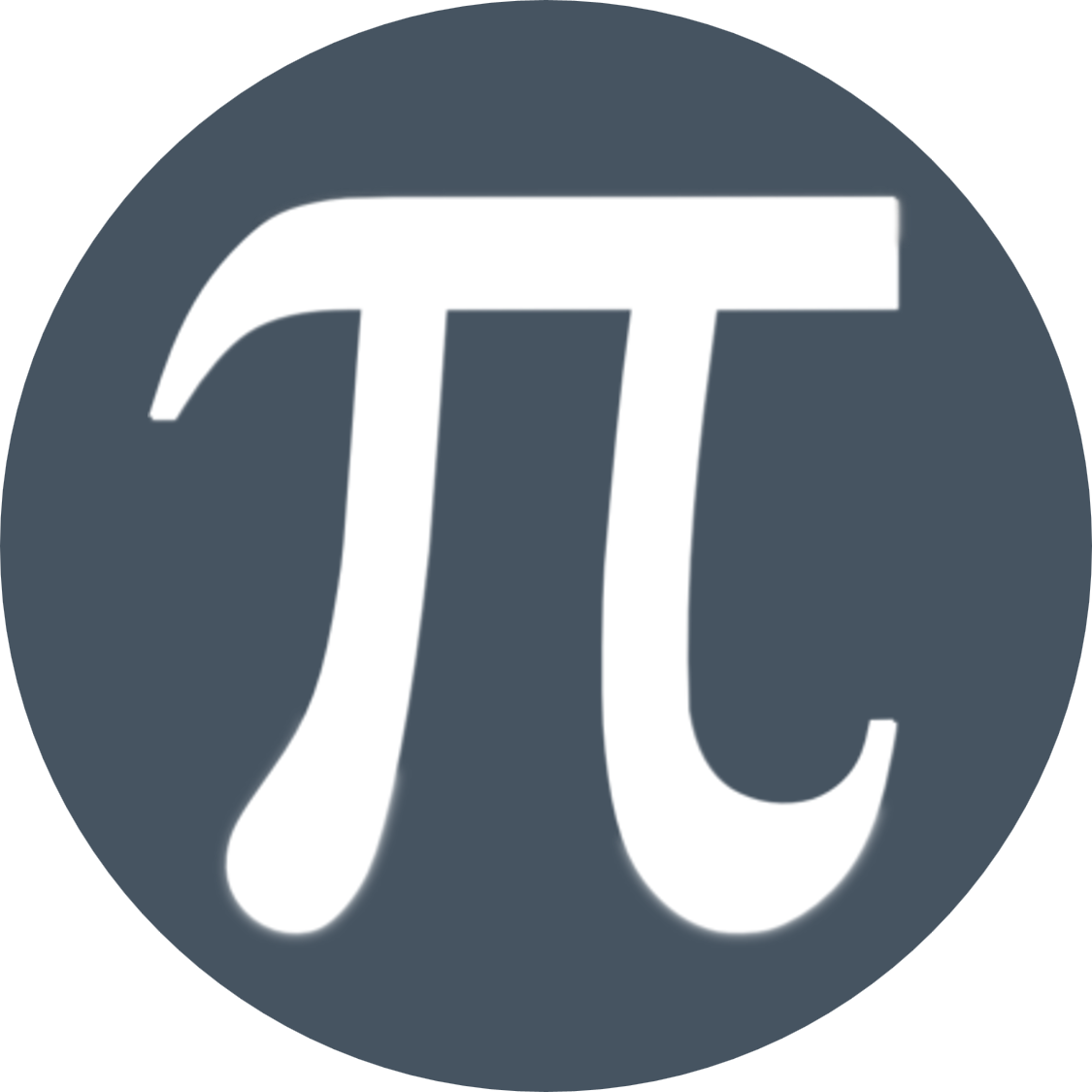# MathematicsMaths is a core subject studied by all students from Y7-11.

You can gain a variety of different skills from maths including problem solving, data analysis, attention to detail and communication skills.

These skills are then applied in a wide range of subjects including Geography, Computing and Science.

Maths is taught in ability groups.  In KS3 You will follow either the 'Foundation' or 'Higher' Curriculum.

## Foundation Curriculum

Year 7 Year 8 Year 9
Aut.1 Number techniques Shape 2D & 3D Symmetries Sequences, Equations & Functions, Statistics
Aut.2 Algebra, Fractions Decimals and Percentages Statistical Measures Scales and Measures
Spr.1 Primes, Factors, Measures, Probability Expressions, Linear equations, & Formulae Pythagoras, Volume, Probability
Spr.2 Angles in Triangles & Quads, Ratio, Fractions Constructions, Number, Basic Pythagoras Venn Diagrams, Real life graphs, independence
Sum.1 Sequences, Perimeter, Area and Volume Experimental Probability Quadratic sequences, Angle geometry
Sum.2 Graphs, Transformations. Straight lines, Functions Loci & Contractions

## Higher Curriculum

Year 7 Year 8 Year 9
Aut.1 Number, Statistical diagrams Shape 2D & 3D, Properties of shapes Equations, Inequalities
Aut.2 Algebra, Fractions Decimals and Percentages Statistical analysis Statistical Analysis and Comparison
Spr.1 Primes, Factors LCM &HCF, Probability Linear & quadratic equations, Formulae Proportion, Arcs and Sectors, Quadratic graphs
Spr.2 Angles in polygons, Ratio and proportion Constructions, Pythagoras, Reasoning Reciprocal graphs, Sequences, Quadratics
Sum.1 Functions, Perimeter, Area and Volume Experimental Probability Quadratic factorising, Circles
Sum.2 Graphs, conversions Transformations Straight lines and perpendiculars Trigonometry in 2D and simple 3D

## Where does this subject lead me?

KS4 Maths

All students study Maths at GCSE.  It is taught in 10 ability sets with students able to move between groups depending on progress.  For info., visit our GCSE Maths page.

Sixth Forms, colleges and employers require either a grade 4 or 5 or above in Mathematics. We guide pupils towards the route that is most likely to deliver this outcome.

The skills taught in Maths are applied in a wide range of our GCSE subjects including:

Career Pathways

There are a shortage of people going into jobs that use lots of maths!

A level Mathematics is a requirement for certain degree courses, such as Accounting, Computing, Product Design, Engineering, Physics, Statistics, and often Economics. Although not a requirement, A level Mathematics is a typical subject taken by students on courses as wide ranging as Architecture, Law and Psychology.

You can watch a great series of videos of young people who are working in jobs that use Maths on the BBC Bitesize website.